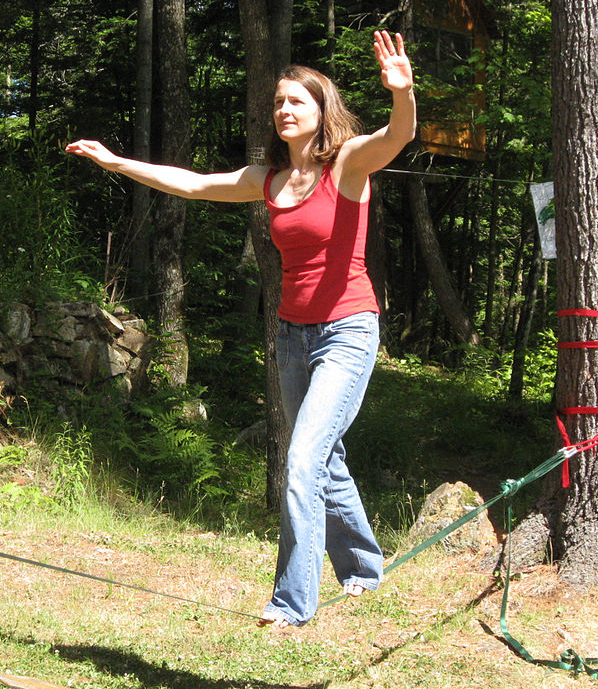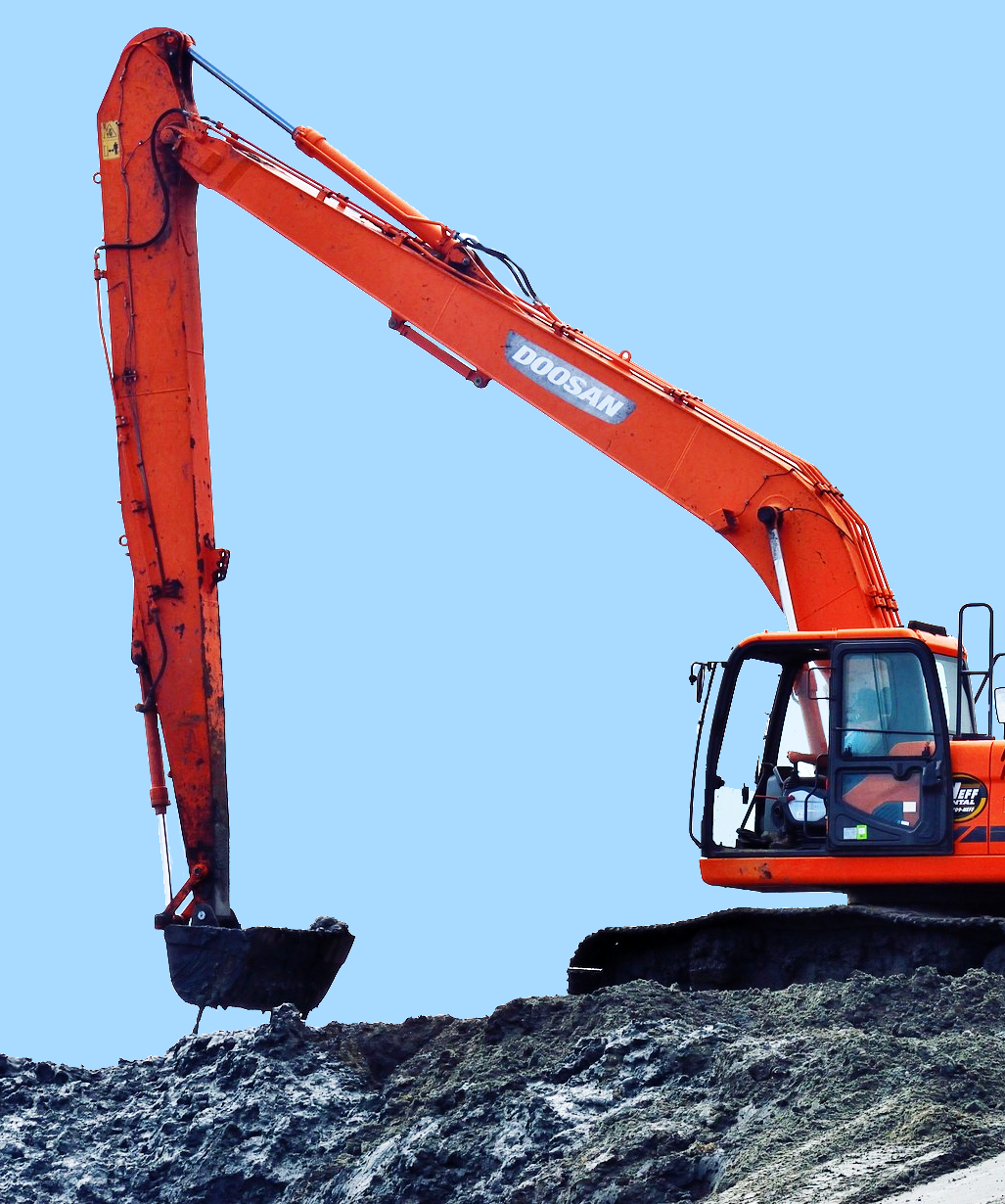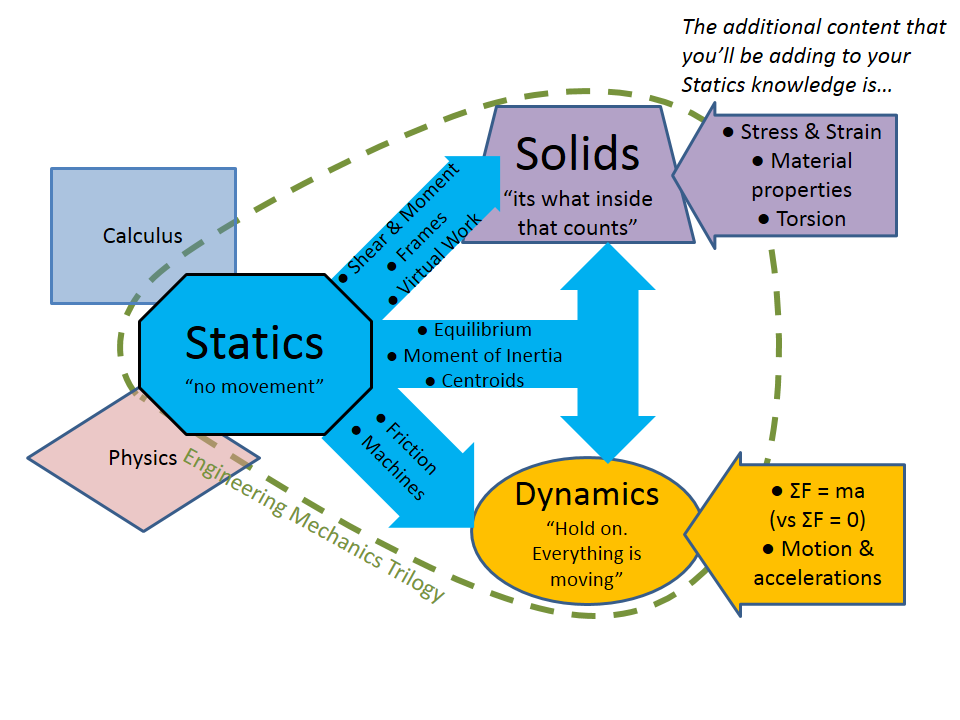# Engineering Statics: Open and Interactive

## Chapter1Introduction to Statics

Engineering Statics is the gateway into engineering mechanics, which is the application of Newtonian physics to design and analyze objects, systems, and structures with respect to motion, deformation, and failure. In addition to learning the subject itself, you will also develop skills in the art and practice of problem solving and mathematical modeling, skills that will benefit you throughout your engineering career.
The subject is called “statics” because it is concerned with particles and rigid bodies that are in equilibrium, and these will usually be stationary, i.e. static.
The chapters in this book are:
Your statics course may not cover all of these topics, or may move through them in a different order.
Below are two examples of the types of problems you’ll learn to solve in statics. Notice that each can be described with a picture and problem statement, a free-body diagram, and equations of equilibrium.
Equilibrium of a particle: A $$\lb{140}$$ person walks across a slackline stretched between two trees. If angles $$\alpha$$ and $$\theta$$ are known, find the tension in each end of the slackline.Person’s point of contact to slackline:
\begin{gather*} \Sigma F_x = 0\\ -T_1 \cos \alpha + T_2 \cos \theta = 0\\ \\ \Sigma F_y = 0\\ T_1 \sin \alpha +T_2 \sin \theta -W = 0 \end{gather*}
Equilibrium of a rigid body: Given the interaction forces at point $$C$$ on the upper arm of the excavator, find the internal axial force, shear force, and bending moment at point $$D\text{.}$$Section cut FBD:
\begin{gather*} \Sigma F_x = 0\\ -C_x + F_x - V_x - N_x = 0\\ \\ \Sigma F_y = 0\\ -C_y - F_y - V_y + N_y = 0\\ \\ \Sigma M_D = 0\\ +(d_y)C_x + (d_x)C_y - M_D = 0 \end{gather*}
The knowledge and skills gained in Statics will be used in your other engineering courses, in particular in Dynamics, Mechanics of Solids (also called Strength or Mechanics of Materials), and in Fluid Mechanics. Statics will be a foundation of your engineering career.Figure 1.0.1. Map of how Statics builds upon the prerequisites of Calculus and Physics and then informs the later courses of Mechanics of Solids and Dynamics.